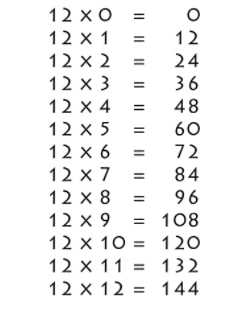# 12 Times Table- Learn Table Of 12 : Multiplication Table Of 12

Safalta expert Published by: Yashaswi More Updated Tue, 07 Jun 2022 11:06 PM IST

## Highlights

Check out how to learn the 12 Times table easily here at Safalta.com

Table of 12: Here are some interesting facts about the number 12. Do you know that till now 12 men have walked on the Moon? Generally, most of us use a 12-hour time system. These facts emphasize the importance of the number 12. Now let us learn about the 12 times table. Table of 12 shows the multiplication of 12 with various natural numbers.

### Free Demo ClassesSource: Safalta.com

Learning a 12 times table gives us an advantage when dealing with problems that involve the multiples of 12. So, let's begin our lesson on the table of 12.  Join Safalta School Online and prepare for Board Exams under the guidance of our expert faculty. Our online school aims to help students prepare for Board Exams by ensuring that students have conceptual clarity in all the subjects and are able to score their maximum in the exams.

1. 12 Times Table Chart
2. Multiplication Table of 12
3. 12 times table
4. Tips for 12 times table
5. Table of 12 upto 20

## 12 Times Table Chart:## Multiplication Table of 12

Learning the multiplication table of 12 is necessary for solving arithmetic problems involving multiples of 12. So let's learn the 12 times table for fast calculations.

## Tips for 12 Times Table

1. 12 doesn’t have any rules that make its multiplication table of 12 easy to memorize, but there is a pattern for every five multiples of twelve: 12, 24, 36, 48, 60, 72, 84, 96, 108, 120

2. The last digit of these multiples always repeat, which means that students can remember these digits to help them with the 12 times table.

## Worksheets on Table of 12

1. ### Example 1: Using 12 times table, evaluate 12 times 10 minus 20.

Solution:

First, we will write 12 times 10 minus 20 mathematically.

Using 12 times table, we have: 12 times 10 minus 20 = 12 × 10 - 20 = 120 - 20 = 100

Thus, 12 times 10 minus 20 is 100.

2. ### Example 2: Using the table of 12, check whether 12 times 6 plus 7 is 80.

Solution:

First, we will write 12 times 6 plus 7 mathematically.

Using table of 12, 12 times 6 plus 7 = 12 × 6 + 7 = 72 + 7 = 79

Thus, 12 times 6 plus 7 is not 80.

3. ### Example 3: A car covers a distance of 12 km with 1 liter of petrol. Evaluate using the table of 12 what distance will it cover with 15 liters of petrol?

Solution:

Distance covered by car with 1 liter of petrol = 12 km

Distance covered by car with 15 liters of petrol = 12 × 15 = 180 km

Thus, the car will cover 180 km with 15 liters of petrol.

## What is the importance of learning Table 2 to 20?

For making mathematical section easier, memorising table 2 to 20 is important.

## How can I learn tables easily?

Daily recite the table as mentioned in the article twice and thrice.

• 12 x 1 = 12.
• 12 x 2 = 24.
• 12 x 3 = 36.
• 12 x 4 = 48.
• 12 x 5 = 60.
• 12 x 6 = 72.
• 12 x 7 = 84.
• 12 x 8 = 96.

## How do I learn 12x?

Adding 12 each time is a common method: 12+12= 24, 24 + 12 = 36, 36+12 = 48. Also notice the pattern in the ones columns: 12 24 36 48 60 72 84 96 108 120 the 0, 2, 4, 6, 8, 0 pattern repeats through all the 12 x tables. You can use the half and double method: So 3 x 12 becomes 6 x 6 = 36.

## What are the multiples of 12?

The multiples of 12 are 12, 24, 36, 48, 60, 72, 84, 96, 108, 120, 132, 144,… The multiples of 16 are 16, 32, 48, 64, 80, 96, 112, 128, 144, 160,… Hence the common multiples of 12 and 16 are 48, 96, 144,… and their LCM is 48.